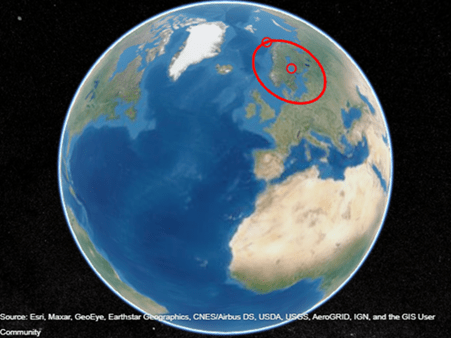# Generate Small Circles

Generate a true small circle, a loxodromic small circle, and the limiting case of a great circle.

Display the map axes with an orthographic projection.

```figure; axesm ortho; gridm on; framem on setm(gca,'Origin', [45 30 30], 'MLineLimit', [75 -75],... 'MLineException',[0 90 180 270])```

Define three center points on the sphere.

```A = [45 90]; B = [0 60]; C = [0 30];```

Create the three small circles around the three center points. The first, `sca`, is a true small circle. The second, `scb`, is a loxodromic small circle. The third, `scc`, is a great circle as the limiting case of a small circle.

```sca = scircle1(A(1), A(2), 20); scb = scircle2(B(1), B(2), 0, 150); scc = scircle1('rh',C(1), C(2), 20);```

Display the points and their corresponding small circles with different colors. Label the small circles.

```plotm(A(1), A(2),'ro','MarkerFaceColor','r') plotm(B(1), B(2),'bo','MarkerFaceColor','b') plotm(C(1), C(2),'mo','MarkerFaceColor','m') plotm(sca(:,1), sca(:,2),'r') plotm(scb(:,1), scb(:,2),'b--') plotm(scc(:,1), scc(:,2),'m') textm(50,0,'Normal Small Circle') textm(46,6,'(20\circ from point A)') textm(4.5,-10,'Loxodromic Small Circle') textm(4,-6,'(20\circ from point C') textm(-2,-4,'in rhumb line sense)') textm(40,-60,'Great Circle as Small Circle') textm(45,-50,'(90\circ from point B)')```